# MP Board Class 6th Maths Solutions Chapter 4 Basic Geometrical Ideas Ex 4.2

## MP Board Class 6th Maths Solutions Chapter 4 Basic Geometrical Ideas Ex 4.2

Question 1.
Classify the following curves as
(i) Open or
(ii) Closed.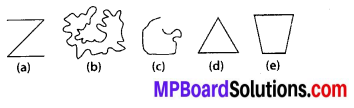Solution:
(a) Open curve
(b) Closed curve
(c) Open curve
(d) Closed curve
(e) Closed curveQuestion 2.
Draw rough diagrams to illustrate the following:
(a) Open curve
(b) Closed curve.
Solution:
(a) Open curves :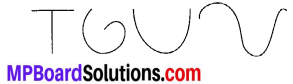(b) Closed curves :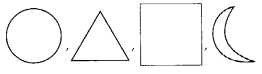Question 3.
Draw any polygon and shade its interior.
Solution: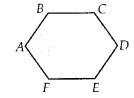ABCDEF is the required polygon.Question 4.
Consider the given figure and answer the questions:
(a) Is it a curve?
(b) Is it closed?
Solution: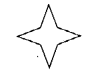(a) Yes, it is a curve.
(b) Yes, it is closed.Question 5.
Illustrate, if possible, each one of the following with a rough diagram:
(a) A closed curve that is not a polygon.
(b) An open curve made up entirely of line segments.
(c) A polygon with two sides.
Solution: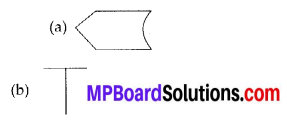(c) Polygon with two sides cannot be drawn.